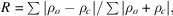International
Tables for
Crystallography
Volume F
Crystallography of biological macromolecules
Edited by M. G. Rossmann and E. Arnold

International Tables for Crystallography (2006). Vol. F. ch. 21.1, p. 505   | 1 | 2 |

## Section 21.1.7.4.2. Real-space fits

G. J. Kleywegta*

aDepartment of Cell and Molecular Biology, Uppsala University, Biomedical Centre, Box 596, SE-751 24 Uppsala, Sweden
Correspondence e-mail: gerard@xray.bmc.uu.se

#### 21.1.7.4.2. Real-space fits

| top | pdf |

The fit of a model to the data can also be assessed in real space, which has the advantage that it can be performed for arbitrary sets of atoms (e.g. for every residue separately). Jones et al. (1991) introduced the real-space R value, which measures the similarity of a map calculated directly from the model (ρ c) and one which incorporates experimental data (ρ o) aswhere the sums extend over all grid points in the map that surround the selected set of atoms. The real-space fit can also be expressed as a correlation coefficient (Jones & Kjeldgaard, 1997), which has the advantage that no scaling of the two densities is necessary. Chapman (1995) described a modification in which the density calculated from the model is derived by Fourier transformation of resolution-truncated atomic scattering factors.

The program SFCHECK (Vaguine et al., 1999) implements several variations on the real-space fit. The normalized average displacement measures the tendency of groups of atoms to move away from their current position. The density correlation is a modification of the real-space correlation coefficient. The residue-density index is calculated as the geometric mean of the density values of a set of atoms, divided by the average density of all atoms in the model. It therefore measures how high the electron-density level is for the set of atoms considered (e.g. all side-chain atoms of a residue). The connectivity index is identical to the residue-density index, but is calculated only for the N, Cα and C atoms. It thus provides an indication of the continuity of the main-chain electron density.

### ReferencesChapman, M. S. (1995). Restrained real-space macromolecular atomic refinement using a new resolution-dependent electron-density function. Acta Cryst. A51, 69–80.Google ScholarJones, T. A. & Kjeldgaard, M. (1997). Electron density map interpretation. Methods Enzymol. 277, 173–208.Google ScholarJones, T. A., Zou, J.-Y., Cowan, S. W. & Kjeldgaard, M. (1991). Improved methods for building protein models in electron density maps and the location of errors in these models. Acta Cryst. A47, 110–119.Google ScholarVaguine, A. A., Richelle, J. & Wodak, S. J. (1999). SFCHECK: a unified set of procedures for evaluating the quality of macromolecular structure-factor data and their agreement with the atomic model. Acta Cryst. D55, 191–205.Google Scholar